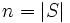# Sign homomorphism on the finitary symmetric group

## Definition for a finite set

Suppose$S$ is a finite set of size at least two. The sign homomorphism, also called the sign map or signum, on the symmetric group$\operatorname{Sym}(S)$ is a homomorphism to the group$\pm 1$ under multiplication, defined as follows. Without loss of generality, assume$S = \{ 1,2,3, \dots n \}$ where$n = |S|$. For a permutation$\sigma$, the sign of$\sigma$ is given by:$\prod_{1 \le i < j \le n} \frac{x_{\sigma(i)} - x_{\sigma(j)}}{x_i - x_j}$.

For$n = 0$ and$n = 1$, the sign homomorphism is defined by fiat to be the trivial map.

The definition is valid because:

• The map does go to$\pm 1$: Both the numerator an denominator are products of the same linear factors, up to a difference of sign.
• The map is a homomorphism: This is easy to check, and follows essentially by cancellation of the denominator in one expression with the numerator in the next.

Further, any transposition (i.e., a permutation that interchanges two elements of$S$ and leaves everything else fixed) has sign$-1$. Thus, if a permutation is expressible as aproduct of$r$ transpositions, its sign is$(-1)^r$.

A permutation whose sign is$+1$ is termed an even permutation and a permutation whose sign is$-1$ is termed an odd permutation. The even permutations form the kernel of the sign homomorphism, and this subgroup of the symmetric group is termed the alternating group.

## Definition for an infinite set

For an infinite set, the sign homomorphism is not defined on the whole symmetric group. Rather, it is defined on the finitary symmetric group. PLACEHOLDER FOR INFORMATION TO BE FILLED IN: [SHOW MORE]